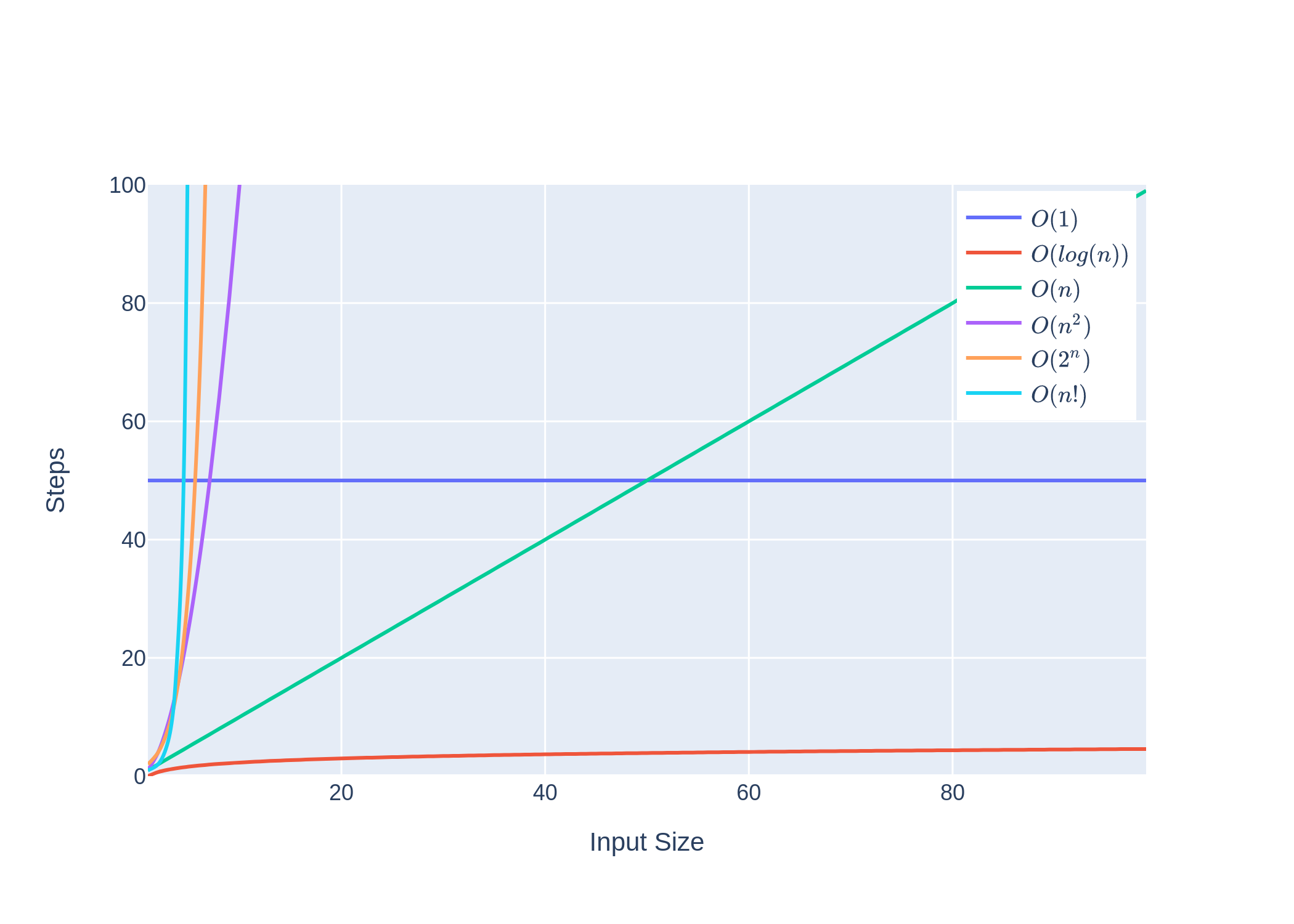# Big-O Notation

Big-O notation is a form of measuring the algorithmic complexity of a function or algorithm in the worst-case scenario. This can either analyze the maximum amount of possible time or memory space needed to solve a particular problem. Solutions can be refactored or reworked to achieve a more efficient time/space complexity with the help of Big-O notation.

## Common Runtimes

Here are some common runtimes:Runtime Name Description Use Case(s)
O(1) Constant time (in blue) The time needed is the same, regardless of the input size. Accessing an element in an array.
O(ln(n)) Logarithmic time (in red) The time is a logarithmic function `ln()` of the input size `n`. Analyzing the binary search algorithm.
O(n) Linear time (in green) The time needed is proportional to the input size `n`. Traversing an array of `n`-size.
O(n^2) Polynomial/quadratic time (in purple) The time needed is the input size, `n`, multiplied by itself. Bubble sort has quadratic time complexity.
O(2^n) Exponential time (in orange) The time needed doubles for every new element added to the input. The Fibonacci Series.
O(n!) Factorial time (in cyan) The time needed is equivalent to the factorial of the input size. The Traveling Salesman problem.

## Simplifying Big-O Expressions

When determining an expression that characterizes the time or space complexity of an algorithm, an expression may contain multiple terms ( e.g., O(n) + O(ln(n)) ). In the context of Big-O, the focus is on the relative change as the input gets large. And as the input gets larger (approaches infinity), the higher-order terms take precedence over the lower-order terms, which is why the Big-O notation would simplify to just O(n).

## Python Big-O Practice

The following Python example analyzes the time/space complexity of the `foo()` function:

```def foo(list1, list2):  for item in list1:    print(f"Outer loop: {item}")    for item2 in list2:      print(f"Inner loop: {item2}")
foo(["Hello", "World"], ["Spam", "Eggs"])
```

The inner loop is equivalent to O(n), and the outer loop will execute the inner loop n times. Therefore, the Big-O notation for the `foo()` function would be n * O(n) = O(n^2).